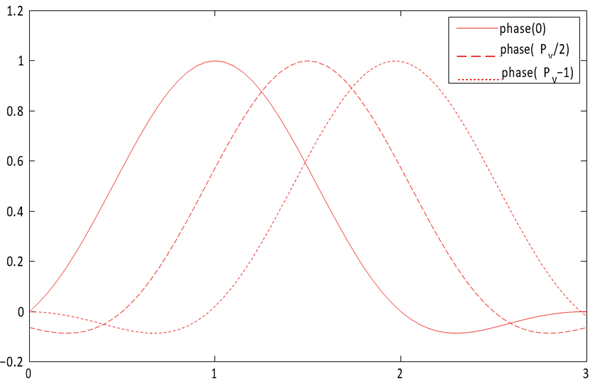ID 683329
Date 12/12/2022
Public

## 29.3.1. Coefficient Selection

If you select polyphase scaling for the Scaler IP, the coefficients that the scaling filters use are read from a memory. You must define the contents of this memory.,Either specify fixed horizontal and vertical coefficient sets in the Horizontal coefficient function and Vertical coefficient function parameters or turn on Update coefficients at runtime. If you turn on Update coefficients at runtime, you can write whatever values you want to the coefficient memory. If you already have well defined coefficient sets that you use, the flexibility in the scaler to select the desired number of filter taps and phases should allow you to continue with these. If you are new to scaling, read the following guidance on coefficient selection.

Generally, the set of coefficients that the IP writes to filter phase 0 yield a low-pass filter, with most weight in generating the output pixel value given to the pixel value in tap . The function is centered at tap . The coefficients the IP writes to the other phases are then just phase-shifted versions of this function (hence the name phase for each coefficient address), with the function centered at a point that is shifted by 1/ num_of_pixels of a pixel with every subsequent phase.

Figure 62. 2-lobe Lanczos function at 3 different phases This figure shows an example of how a function is progressively phase-shifted to create the coefficients for each scaling filter phase. The figure shows a 4 tap filter, with the taps shown on the x-axisThe Lanczos function is a common function that defines scaling coefficients. The Lanczos function is a base sinc function, with the primary lobe of a sinc function used as a window function to preserve a given number of lobes of the base sinc. The number of lobes is generally appended to Lanczos when referring to a specific variant of the function, so a Lanczos function where two lobes are preserved is referred to as Lanczos2. In the case where N lobes are preserved, the LanczosN function is defined as:

Equation 8. Coefficient Selection Equation

When using LanczosN coefficients, Intel recommends configuring the scaler filters with the following numbers of taps for the upscale and downscale cases:

• Upscale: 2 × N
• Downscale:

The number of lobes in the Lanczos function affects the frequency response of the filter and, as a result, the quality of the image produced. Generally, Lanczos functions with lower numbers of lobes give a softer frequency response and a resulting image with more blur on the edges, but with less risk of ringing artifacts in the areas immediately around the edges. Conversely, Lanczos functions with higher numbers of lobes give sharper edges but introduce more ringing artifacts. Lanczos2 is a good compromise between minimizing blur and minimizing ringing, but you can experiment with Lanczos3 or Lanczos4 to make your own judgment. The higher the number of lobes, the more filter taps (and therefore FPGA device resources) the design requires to implement the filter correctly. You should experiment with Lanczos1 coefficients for large downscales.